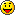How to get position just behind car.I need X,Y and Z.
I have X Y Z of green Car
Assume that coordinate for green car is X1, Y1 and Z1
So what will the possible coordinates of Brown car.
hmm use debug type /vsel and press at sultan then park it use /save greencar after do again /vsel choose sultan then park behind greencar /save browncar after go at documents Gta then SAMP >>>Savepositions and copy the coordinations and doneif you mean for this
Quote:
 Originally Posted by Diti1hmm use debug type /vsel and press at sultan then park it use /save greencar after do again /vsel choose sultan then park behind greencar /save browncar after go at documents Gta then SAMP >>>Savepositions and copy the coordinations and doneif you mean for this
I need it dynamically.
Create a command with sscanf (something like createcarnearcar [carid]) and after checking if the carid given is exist, get the vehicle position of that carid and use CreateVehicle, decrease the Y by 3 (Y-3) or more if needed and it should work fine.
Use that with a negative distance and the z angle of the vehicle
Or add / subtract 180 degrees to the angle and use a positive distance
pawn Код:
`stock GetXYFromAngle(Float: angle, Float:distance, & Float: x, & Float: y) {    x -= (distance * floatsin(angle, degrees));    y += (distance * floatcos(angle, degrees));}`
Thats trigonometry. Im not sure about the exact angles as SA-MP angles always behave strange.

pawn Код:
`//new Float:x, Float:y, Float:z, Float:rot;    // is Vehicle 1 position and rotationnew Float:cx, Float:cy, Float:cz; // is Vehicle 2 positioncx = x + floatsin(360.0 - (rot + 180.0), degrees) * distance_between_vehicles;    // "360.0 -" is to transform the samp angle to the mathematical onecy = y + floatcos(360.0 - (rot + 180.0), degrees) * distance_between_vehicles;  //  "+ 180.0" is to point behind the other vehicle, else it would spawn in front of it`
Thats a pretty common question, you could have searchedQuote:
 Originally Posted by MauzenThats trigonometry. Im not sure about the exact angles as SA-MP angles always behave strange. pawn Код: `//new Float:x, Float:y, Float:z, Float:rot;    // is Vehicle 1 position and rotationnew Float:cx, Float:cy, Float:cz; // is Vehicle 2 positioncx = x + floatsin(360.0 - (rot + 180.0), degrees) * distance_between_vehicles;    // "360.0 -" is to transform the samp angle to the mathematical onecy = y + floatcos(360.0 - (rot + 180.0), degrees) * distance_between_vehicles;  //  "+ 180.0" is to point behind the other vehicle, else it would spawn in front of it` Thats a pretty common question, you could have searchedThanksYou earned my respect sir. [Not Forum Respect]Forum Jump:

Users browsing this thread: 1 Guest(s)